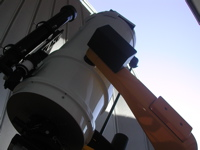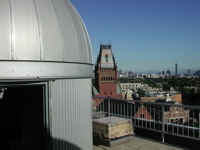# Astronomy Lab and Clay TelescopeThe Measurement of the Astronomical Unit - Final Report

SPU-21 Fall 2015

printer version

For X = Y/Z; with uncertainties in Y and Z with values uy, uz, then uncertainties in X, ux, is given (ux/X)2 = (uy/Y)2 + (uz/Z)2 so that the uncertainty on the ratio, X, is then ux = X √[(uy/Y2 + (uz/Z)2]

This is not an easy measurement in Astronomy but one that can be done from our lab in Cambridge, MA. Refer back to the above labs to remind yourself of how you obtained these measurements.

Format:

Introduction: Motivation (~0.5 page) to measure AU and Rsun and so Lsun, given Tsun? What is AU so fundamental?

Observations: Briefly describe (~1-2 pages) the observations you did for your ObsReports 1-4 and your observations that led to your estimate of the measurement uncertainties - e.g., your repeated pinhole image diameter measurements; time for Sun to move its diameter measures; and how you estimated then in the Sun's angular diameter As. Similarly, the uncertainties in your Psyn value given the number of sunspots you measured and their likely scatter in solar latitude; and finally your measure of V: how stable was the setup (i.e. did the relluric line(s) stay "fixed" and if not, how much did they move and did you account for this [i.e. subtract off that shift] in deriving your value for V?

Data Reduction: Brief summary (~1-2 pages) of how you "reduced" your measured observations and derived your measurement uncertainties. Make a Table with your resulting measured quantities Rs, As, V, and P and your estimate of their uncertainties.

Data Analysis: In this section (~1.5 pages), you describe how you used your measurements to derive the value of the AU and what its estimated uncertainty is. So this is where you use the formula above for the uncertainty from a ratio (to derive your 2 measures of angular diameter). Estimate your errors, both measured and systematic, in your values for Psyn and thus Psid (you don't need to wrorry about error in Pearth!) and in your measured value for V.

Discussion: In this section (~1 page), you discuss the overall measurement of the AU and how well your final value compares with the actual AU (given above). You can also then discuss why the AU is such an important number to measure the distance to star (e.g. first rung on the distance ladder is?) and what you can then "measure" from it - e.g. mass of Sun, distance to planets (e.g. Mars) given their orbital period, distance to the star Vega (at 9pc distance) in km units, or mass of our entire Milky Way Galaxy by measures of its rotation curve from the velocities of stars in our Galactic Plane at a "measured" distances d (in pc) and angular offsets, L given by their galatic longitude values. Do this for a single star at longitude L = 90° (for a star like Deneb!). So consider what your AU allows...!

Figures and references: Include figures (hand-drawn sketches are fine!) to make it clear what your are describing, with Fig. numbers. If you use "outside" information, be sure to include references, as you would with any paper. Please include your "worksheets" showing derivations as an Apprendix at the end of the Report.